# Public Key Cryptography Department of Computer Science and

• Slides: 18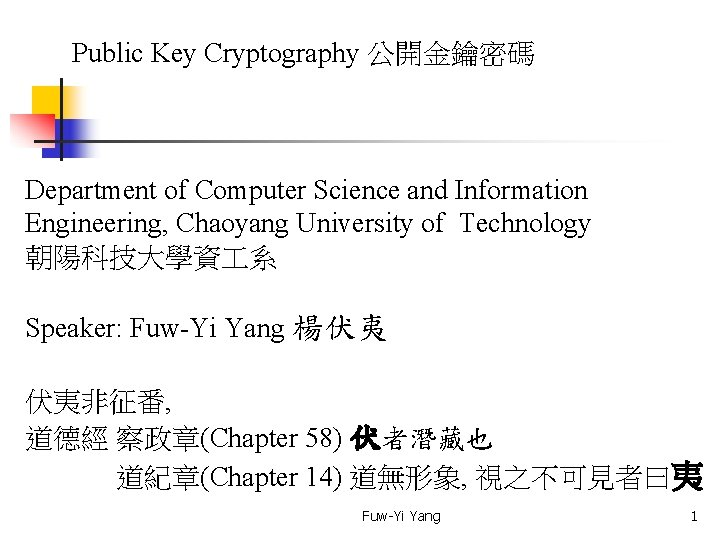Public Key Cryptography 公開金鑰密碼 Department of Computer Science and Information Engineering, Chaoyang University of Technology 朝陽科技大學資 系 Speaker: Fuw-Yi Yang 楊伏夷 伏夷非征番, 道德經 察政章(Chapter 58) 伏者潛藏也 道紀章(Chapter 14) 道無形象, 視之不可見者曰夷 Fuw-Yi Yang 1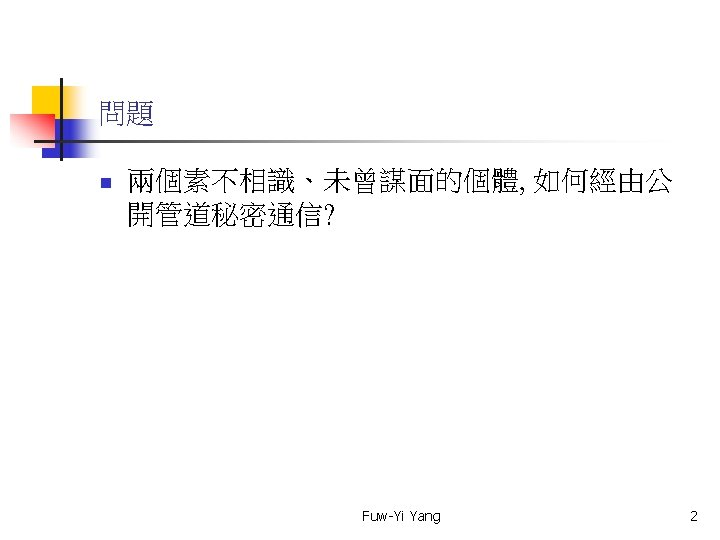RSA Public Key Cryptosystem 1/3 RSA 公開金鑰密碼系統 ㄚ蜜的 公開金鑰: n = 77, e = 7 (私藏秘密金鑰 d = 43) 任何人寄資料(資料 m = 5)給ㄚ蜜: 密文 c = me mod 77 = 5 7 mod 77 = 5 5 mod 77 = 125 5 125 mod 77 = 48 5 48 mod 77 = 9 48 mod 77 = 47 將密文 c = 47 經由公開網路傳遞給ㄚ蜜 Fuw-Yi Yang 3RSA Public Key Cryptosystem 2/3 RSA 公開金鑰密碼系統 ㄚ蜜的 公開金鑰: n = 77, e = 7 (私藏秘密金鑰 d = 43) 當ㄚ蜜收到密文 c 時, 解密: 密文 c = 47 資料 m = cd mod 77 = 4743 mod 77 = 47 … 47 mod 77 = 23 53 47 mod 77 =5 Note: 23 =4710 mod 77, 53 =4732 mod 77 Fuw-Yi Yang 4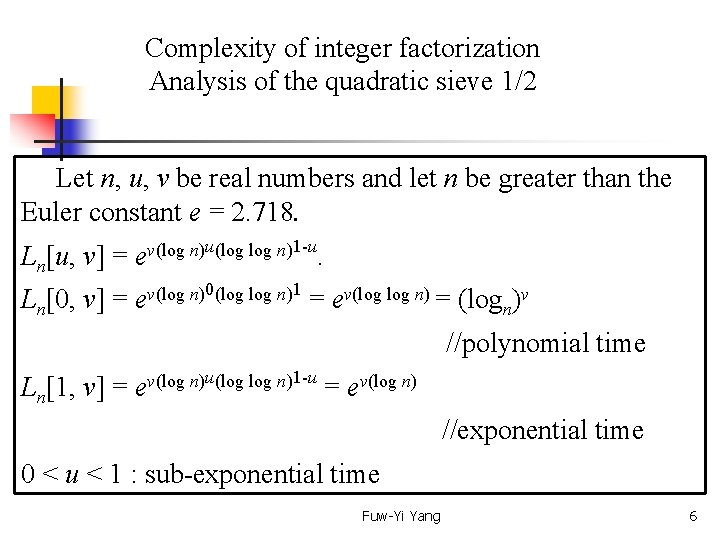Complexity of integer factorization Analysis of the quadratic sieve 1/2 Let n, u, v be real numbers and let n be greater than the Euler constant e = 2. 718. Ln[u, v] = u(log n)1 -u v(log n) e. Ln[0, v] = 0(log n)1 v(log n) e = ev(log n) = (logn)v //polynomial time Ln[1, v] = ev(log n)u(log n)1 -u = ev(log n) //exponential time 0 < u < 1 : sub-exponential time Fuw-Yi Yang 6Complexity of integer factorization Analysis of the quadratic sieve 2/2 In 1988, John Pollard invented the number field sieve (NFS). Under appropriate assumptions, it can be shown that the running time of NFS is Ln[1/3, (64/9)1/3] = Ln[1/3, 1. 923] = e 1. 923(log n)1/3(log n)2/3 For n = 21024, log n = loge n = 709. 78 Ln[1/3, 1. 923] = e 1. 923*8. 92*(6. 56)2/3 = e 1. 923*8. 92*3. 5 = e 60. 1 ≒ 286≒ 1025. 9 Fuw-Yi Yang 7Complexity of Discrete logarithm problem Analysis of the Pollard ρ -Algorithm Discrete Logarithm Problem (DLP): G is a finite cyclic of order n, g is a generator of this group, and 1 is the neutral element of G. Let y be an element randomly selected from G. DLP is to find an integer 0 ≦ x ≦ n such that gx = y. Algorithms: baby-step giant-step, Pollard Time complexity: |G|1/2 Fuw-Yi Yang 8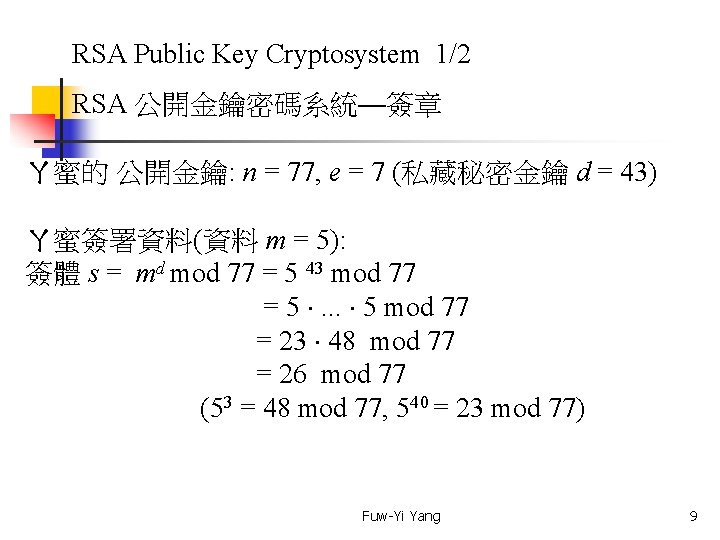RSA Public Key Cryptosystem 1/2 RSA 公開金鑰密碼系統—簽章 ㄚ蜜的 公開金鑰: n = 77, e = 7 (私藏秘密金鑰 d = 43) ㄚ蜜簽署資料(資料 m = 5): 簽體 s = md mod 77 = 5 43 mod 77 = 5 . . . 5 mod 77 = 23 48 mod 77 = 26 mod 77 (53 = 48 mod 77, 540 = 23 mod 77) Fuw-Yi Yang 9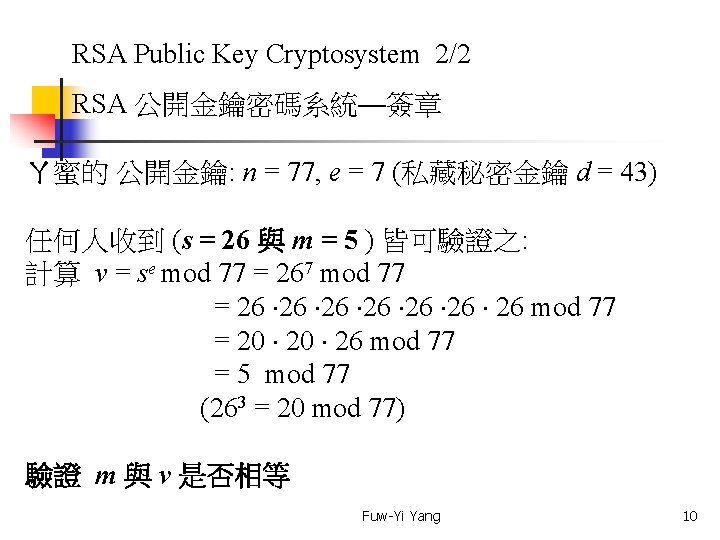RSA Public Key Cryptosystem 2/2 RSA 公開金鑰密碼系統—簽章 ㄚ蜜的 公開金鑰: n = 77, e = 7 (私藏秘密金鑰 d = 43) 任何人收到 (s = 26 與 m = 5 ) 皆可驗證之: 計算 v = se mod 77 = 267 mod 77 = 26 26 26 26 mod 77 = 20 26 mod 77 = 5 mod 77 (263 = 20 mod 77) 驗證 m 與 v 是否相等 Fuw-Yi Yang 10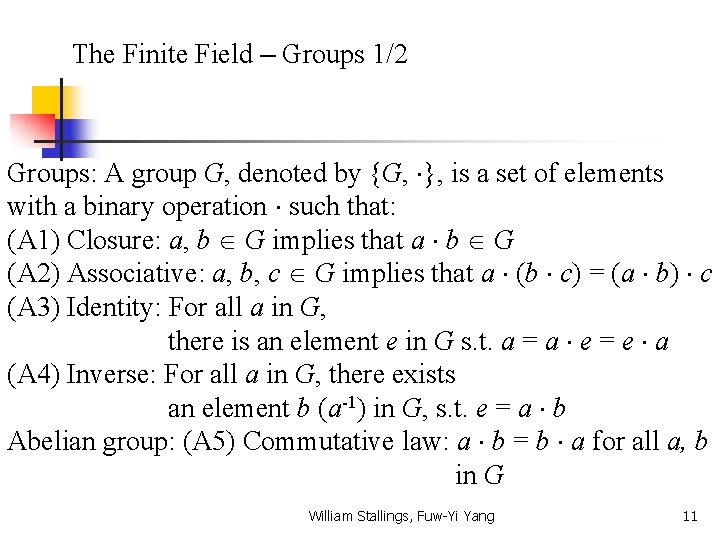The Finite Field – Groups 1/2 Groups: A group G, denoted by {G, }, is a set of elements with a binary operation such that: (A 1) Closure: a, b G implies that a b G (A 2) Associative: a, b, c G implies that a (b c) = (a b) c (A 3) Identity: For all a in G, there is an element e in G s. t. a = a e = e a (A 4) Inverse: For all a in G, there exists an element b (a-1) in G, s. t. e = a b Abelian group: (A 5) Commutative law: a b = b a for all a, b in G William Stallings, Fuw-Yi Yang 11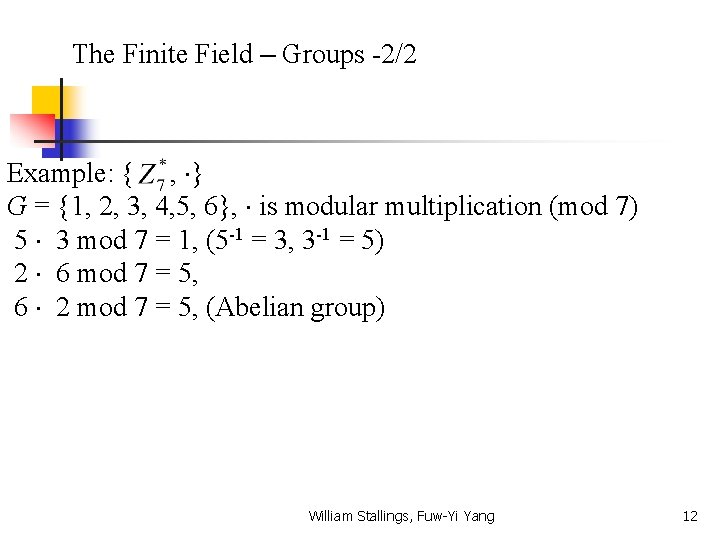The Finite Field – Groups -2/2 Example: { , } G = {1, 2, 3, 4, 5, 6}, is modular multiplication (mod 7) 5 3 mod 7 = 1, (5 -1 = 3, 3 -1 = 5) 2 6 mod 7 = 5, 6 2 mod 7 = 5, (Abelian group) William Stallings, Fuw-Yi Yang 12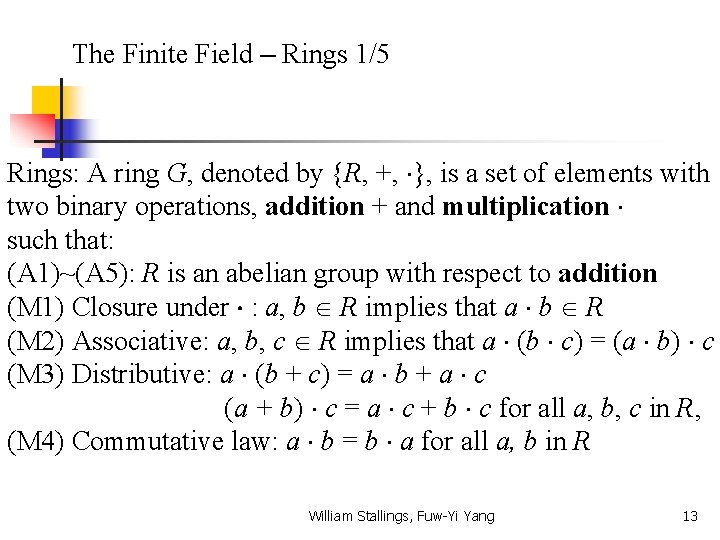The Finite Field – Rings 1/5 Rings: A ring G, denoted by {R, +, }, is a set of elements with two binary operations, addition + and multiplication such that: (A 1)~(A 5): R is an abelian group with respect to addition (M 1) Closure under : a, b R implies that a b R (M 2) Associative: a, b, c R implies that a (b c) = (a b) c (M 3) Distributive: a (b + c) = a b + a c (a + b) c = a c + b c for all a, b, c in R, (M 4) Commutative law: a b = b a for all a, b in R William Stallings, Fuw-Yi Yang 13The Finite Field – Rings (integral domain) 2/5 Integral Domain: (M 5) Multiplicative identity: there is an element 1 in R s. t. a = a 1 = 1 a (M 6) No zero divisors: If a, b in R and a b = 0, then either a = 0 or b = 0 William Stallings, Fuw-Yi Yang 14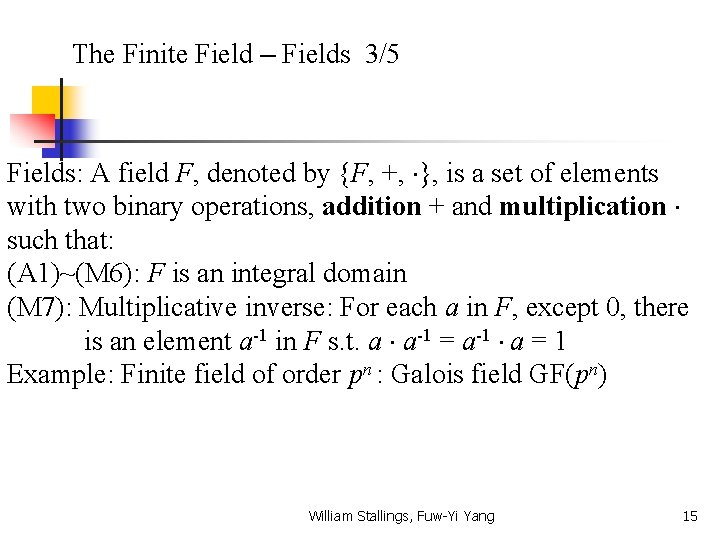The Finite Field – Fields 3/5 Fields: A field F, denoted by {F, +, }, is a set of elements with two binary operations, addition + and multiplication such that: (A 1)~(M 6): F is an integral domain (M 7): Multiplicative inverse: For each a in F, except 0, there is an element a-1 in F s. t. a a-1 = a-1 a = 1 Example: Finite field of order pn : Galois field GF(pn) William Stallings, Fuw-Yi Yang 15The Finite Field – GF(7)-addition 4/5 modulo 7 + 0 1 2 3 4 5 6 0 0 1 2 3 4 5 6 1 1 2 3 4 5 6 0 2 2 3 4 5 6 0 1 3 3 4 5 6 0 1 2 4 4 5 6 0 1 2 3 5 5 6 0 1 2 3 4 William Stallings, Fuw-Yi Yang 6 6 0 1 2 3 4 5 16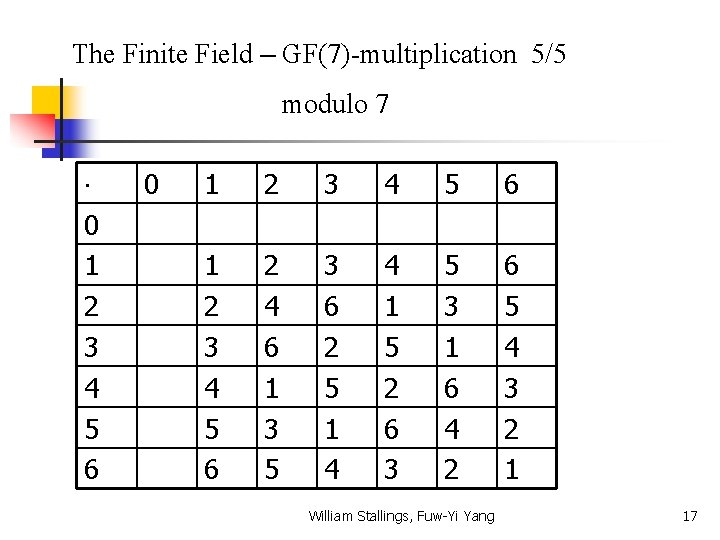The Finite Field – GF(7)-multiplication 5/5 modulo 7 0 1 2 3 4 5 6 2 4 6 1 3 5 3 6 2 5 1 4 4 1 5 2 6 3 5 3 1 6 4 2 6 5 4 3 2 1 William Stallings, Fuw-Yi Yang 17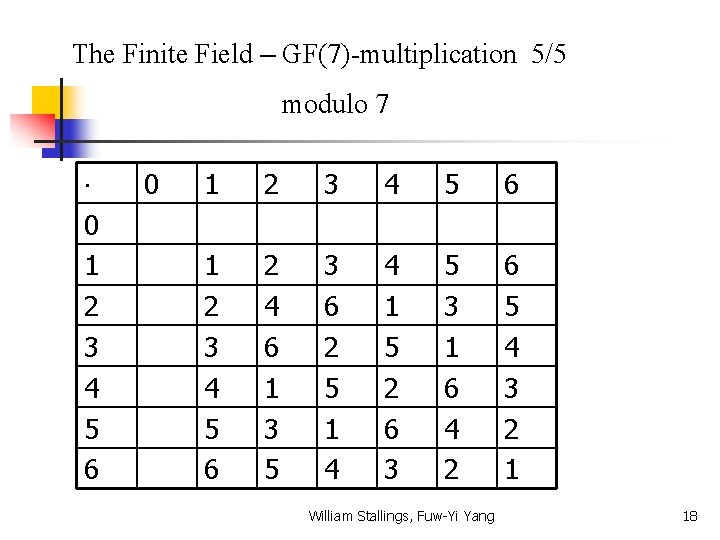The Finite Field – GF(7)-multiplication 5/5 modulo 7 0 1 2 3 4 5 6 2 4 6 1 3 5 3 6 2 5 1 4 4 1 5 2 6 3 5 3 1 6 4 2 6 5 4 3 2 1 William Stallings, Fuw-Yi Yang 18